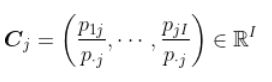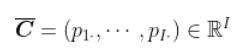# R语言 PCA(主成分分析)，CA(对应分析)夫妻职业差异和马赛克图可视化

PCA(主成分分析)是Pearson在1901年提出的,再后来由hotelling在1933年加以发展提出的一种多变量的统计方法，其最主要的用途在于“降维”，通过析取主成分显出的最大的个别差异,也可以用来削减回归分析和聚类分析中变量的数目，与因子分析类似。

# 夫妻职业数据

``read.table(data.csv",header=TRUE)``

``chisq.test(M)``

# 马赛克图

Mosaic plot常常用来展示Categorical data(分类数据)(关于不同的数据类别，mosaic plot 强大的地方在于它能够很好的展示出2个或者多个分类型变量(categorical variable)的关系. 它也可以定义为用图像的方式展示分类型数据。

``plot(tM)``

``plot(M)``

# 主成分分析和对应分析

### 随时关注您喜欢的主题

``N/apply(N,1,sum)``

``L0=(t(L)-Lbar)``

``PCA(L0,scal=FALSE``

# 我们的想法是将对应于行的个体进行可视化。在第二步中，我们做相同的事情，在列中``N/apply(N,2,sum))````C0=C-Cbar``

# 主成分分析

``PCA(matC0``

# 对应分析

``> plot(C\[,1:2\])``

``> afc=CA(N)``

Kaizong Ye拓端研究室（TRL）的研究员。

​非常感谢您阅读本文，如需帮助请联系我们！QQ在线咨询

15121130882

0571-63341498

## 关注有关新文章的微信公众号

This will close in 0 seconds